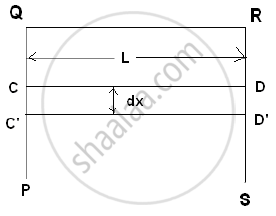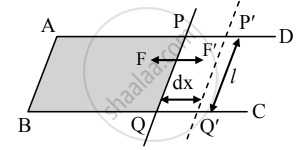# Derive the Relation Between Surface Tension and Surface Energy per Unit Area. - Physics

Derivation
Sum

Derive the relation between surface tension and surface energy per unit area.

Derive the relation between surface energy & surface tension.

#### Solution 1

Surface tension tries to decrease the surface area of a liquid. For increasing surface area, the work has to be done against the surface tension and it is stored in the surface molecules in the form of potential energyConsider a rectangular frame PQRS having a movable wire CD. Let QR = CD = L. If a soap film is formed on the frame CQRD, then the surface tension will try to pull the
wire inward by a force F . "Surface tension" = "Force"/"free Length"

F = Surface tension x Free length

∴ F = T (2L)
If the wire is pulled out to C'D' through distance ‘dx’
∴ Work done = F. dx
∴ W = T (2Ldx)
∴W = T (2Ldx)
But increase in area = dA = 2Ldx
Surface energy is defined as the work done per unit area to increase the free surface area, under isothermal condition.

therefore "Surface energy"="Work done"/"Free surface area"=W/"dA"=(T(2Ldx))/(2Ldx)=T

∴ Surface energy is equal to surface tension

#### Solution 2

The relation between surface tension and surface energy:

1. Let ABCD be a rectangular frame of wire, fitted with a movable arm PQ.2. The frame held in a horizontal position is dipped into a soap solution and taken out so that a soap film APQB is formed. Due to the surface tension of the soap solution, a force ‘F’ will act on each arm of the frame. Under the action of this force, the movable arm PQ moves towards AB.
3. The magnitude of force due to surface tension is, F = 2Tl .....[∵ T = F/l]
(A factor of 2 appears because soap film has two surfaces that are in contact with a wire.)
4. Let the wire PQ be pulled outwards through a small distance ‘dx’ to the position P′Q′, by applying an external force F′ isothermally, which is equal and opposite to F. Work done by this force, dW = F′dx = 2Tldx.
5. But, 2ldx = dA = increase in the area of two surfaces of the film.
∴ dW = T dA
6. This work done in stretching the film is stored in the area dA in the form of potential energy (surface energy).
∴ Surface energy, E = T dA
∴ E/(dA) = T
Hence, surface tension = surface energy per unit area.
7. Thus, surface tension is equal to the mechanical work done per unit surface area of the liquid, which is also called surface energy.
Concept: Surface Tension
Is there an error in this question or solution?
Chapter 2: Mechanical Properties of fluids - Long Answer

Share